# Direction field

(diff) ← Older revision | Latest revision (diff) | Newer revision → (diff)

A geometrical interpretation of the set of line elements corresponding to a system of ordinary differential equations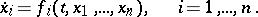(1)

A line element is defined as a sequence of numbers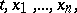(2)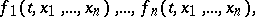where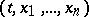is a point of the domain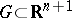, at which the terms on the right-hand side of (1) are defined. A line element (2) can be described as a point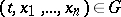together with the direction with direction cosines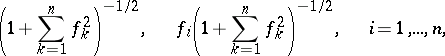(3)

which is represented by a short segment through the point, parallel to the vector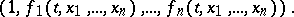For a system in symmetric form,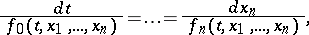unlike system (1), the field may also contain directions orthogonal to the-axis.

Consider any integral curve of the system (1). At every point of this curve, the direction field corresponding to the point is tangent to the curve at that point; any curve with this property is an integral curve of (1). Thus, the specification of a direction field is equivalent to the specification of the system (1), and the problem of integrating this system amounts to the determination of the curves in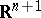the tangents of which at each point possess the directions defined by the formulas (3), i.e. the directions coinciding with the directions of the field at that point.

The geometrical interpretation is particularly easy to visualize when. In that case, for each point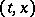of the domain of definition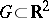of the right-hand side in the first-order equation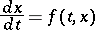(4)

there exists a short segment through the point, with slope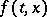, such that the (directed) angle between the-axis and the segment is equal to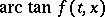(see Fig.).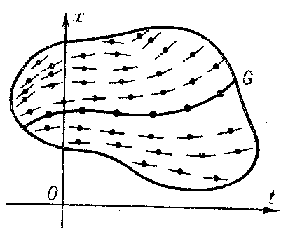Figure: d032780a

Frequently, one considers equation (4) together with the differential equation(5)

wherefor points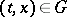at which, defining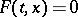for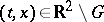, provided this definition preserves the continuity of. By this device, the domainfor the pair of equations (4), (5) is extended to a domain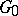by the addition of the points at which the direction is parallel to the-axis, and the integral curves are also allowed to have points with vertical tangents.

If the direction field is drawn in sufficient detail infor equation (4) (or infor the pair of equations (4), (5)), the pattern of line segments will provide a rough qualitative idea of the behaviour of the integral curves. This idea is the basis for the approximate graphical method for solving equation (4), known as the method of isoclines, in which the direction field is constructed with the aid of isoclines (cf. Isocline). The geometrical relationship between the direction field and the integral curves is also the basis for an approximate numerical method for solving equation (4) — the Euler method.

For autonomous systems (cf. Autonomous system) of ordinary differential equations there is a more convenient and intuitive geometrical interpretation in terms of a vector field (cf. also Vector field on a manifold) — the field of phase velocities in the phase space of the system.

How to Cite This Entry:
Direction field. Encyclopedia of Mathematics. URL: http://encyclopediaofmath.org/index.php?title=Direction_field&oldid=12439
This article was adapted from an original article by N.Kh. Rozov (originator), which appeared in Encyclopedia of Mathematics - ISBN 1402006098. See original article